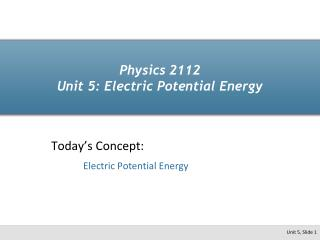DownloadDownload PresentationPhysics 2112 Unit 5: Electric Potential Energy

# Physics 2112 Unit 5: Electric Potential Energy

Download Presentation## Physics 2112 Unit 5: Electric Potential Energy

- - - - - - - - - - - - - - - - - - - - - - - - - - - E N D - - - - - - - - - - - - - - - - - - - - - - - - - - -
##### Presentation Transcript

1. Physics 2112Unit 5: Electric Potential Energy Today’s Concept: Electric Potential Energy

2. Where we’re headed…. Force Energy 2111 2112 ??? E Field

3. Recall from Mechanics: F W>0 Object speeds up (DK>0) dr F dr or W<0 Object slows down ( DK < 0) F dr F W=0 Constant speed ( DK=0) dr

4. Prelecture Question Masses M1 and M2 are initially separated by a distance Ra. Mass M2 is now moved further away from mass M1 such that their final separation is Rb. Which of the following statements best describes the work Wab done by the force of gravity on M2 as it moves from Ra to Rb? Wab > 0 Wab = 0 Wab < 0

5. Potential Energy F Dx If gravity does negative work, potential energy increases! Same idea for Coulomb force… if Coulomb force does negative work, potential energy increases. + + Coulomb force does negative work Potential energy increases + +

6. CheckPoint: Motion of Point Charge Electric Field F Dx A charge is released from rest in a region of electric field. The charge will start to move A) In a direction that makes its potential energy increase.B) In a direction that makes its potential energy decrease.C) Along a path of constant potential energy. It will move in the same direction as F Work done by force is positive DU= -Work is negative Nature wants things to move in such a way that PE decreases

7. Example: Two Point Charges Calculate the change in potential energy for two point charges originally very far apart moved to a separation of “d” d q1 q2 (Charged particles with the same sign have an increase in potential energy when brought closer together.) For point charges often choose r= infinity as “zero” potential energy.

8. Example 5.1 (Velocity after a long time) The two charges shown below are held in place and then released. What is their final velocity after they have q1 d = 10cm q2 m1 = 5X10-10kg q1 = +6nC m2 = 7X10-10kg q2 = +8nC

9. CheckPoint: EPE of Point Charge A charge of +Q is fixed in space. A second charge of +q was first placed at a distance r1 away from +Q. Then it was moved along a straight line to a new position at a distance R away from its starting position. The final location of +q is at a distance r2 from +Q. What is the change in the potential energy of charge +q during this process? • kQq/R • kQqR/r12 • kQqR/r22 • kQq((1/r2)-(1/r1)) • kQq((1/r1)-(1/r2))

10. Potential Energy of Many Charges d d Two charges are separated by a distance d. What is the change in potential energy when a third charge q is brought from far away to a distance d from the original two charges? Q1 Q2 q d (superposition) • Don’t “double count” • No sines and cosines

11. CheckPoint: EPE of a System of Point Charges 1 Two charges which are equal in magnitude, but opposite in sign, are placed at equal distances from point A as shown. If a third charge is added to the system and placed at point A, how does the electric potential energy of the charge collection change? • Potential energy increases • Potential energy decreases • Potential energy does not change • The answer depends on the sign of the third charge

12. CheckPoint: EPE of a System of Point Charges 2 Two point charges are separated by some distance as shown. The charge of the first is positive. The charge of the second is negative and its magnitude is twice as large as the first. Is it possible find a place to bring a third charge in from infinity without changing the total potential energy of the system? YES, as long as the third charge is positive YES, as long as the third charge is negative YES, no matter what the sign of the third charge NO

13. Summary For a pair of charges: r Just evaluate (We usually choose U = 0 to be where the charges are far apart) Q1 Q2 For a collection of charges: for all pairs Sum up